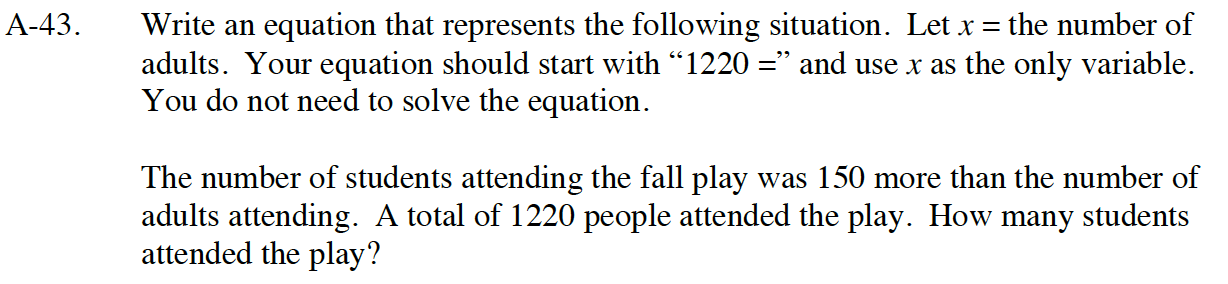Home > CC4 > Chapter A > Lesson A.1.4 > ProblemA-43

A-43.

Write an equation that represents the following situation. Let x = the number of adults. Your equation should start with “1220 =” and use x as the only variable. You do not need to solve the equation.

The number of students attending the fall play was 150 more than the number of adults attending. A total of 1220 people attended the play. How many students attended the play? Homework Help ✎Start by writing an expression that gives the number of students in terms of the number of adults who attended.

x + 150

The total number of people who attended the play is all of the adults plus all of the students.

Be sure to answer the question that the problem asks.

685 students